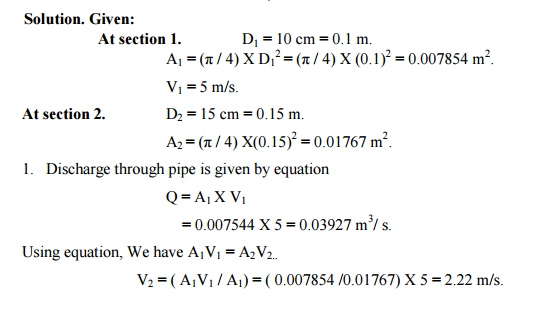# The diameters of a pipe at the sections 1 and 2 are 10 cm and 15 cm respectively. Find the discharge through the pipe if the velocity of water flowing through the pipe section 1 is 5 m/s. determine also the velocity at section 2.

Question-AnswerCategory: Fluid MechanicsThe diameters of a pipe at the sections 1 and 2 are 10 cm and 15 cm respectively. Find the discharge through the pipe if the velocity of water flowing through the pipe section 1 is 5 m/s. determine also the velocity at section 2.

The diameters of a pipe at the sections 1 and 2 are 10 cm and 15 cm respectively. Find the discharge through the pipe if the velocity of water flowing through the pipe section 1 is 5 m/s. determine also the velocity at section 2.